# Doc: Basics Concepts - Inverse Trigonometric Functions JEE Notes | EduRev

## JEE : Doc: Basics Concepts - Inverse Trigonometric Functions JEE Notes | EduRev

The document Doc: Basics Concepts - Inverse Trigonometric Functions JEE Notes | EduRev is a part of the JEE Course Mathematics (Maths) Class 12.
All you need of JEE at this link: JEE

What is an Inverse Function?

f y=f(x) and x=g(y) are two functions such that f (g(y)) = y and g (f(y)) = x, then f and y are said to be inverse of each other i.e.,

g = f-1

If y = f(x) then x = f-1 (y)

Inverse Trigonometric Formulas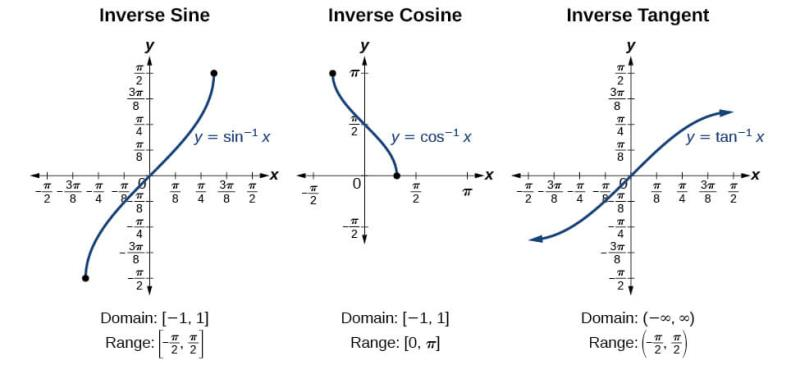Fig: Inverse Trigonometric Functions

The inverse trigonometric functions are the inverse functions of the trigonometric functions written as cos-1 x, sin-1 x, tan-1 x, cot-1 x, cosec-1 x, sec-1 x.

The inverse trigonometric functions are multivalued. For example, there are multiple values of ω such that z = sinω, so sin-1z is not uniquely defined unless a principal value is defined.

Such principal values are sometimes denoted with a capital letter so, for example, the principal value of the inverse sine may be variously denoted sin-1z or arcsinz.

Let’s say, if y = sin x , then x = sin-1 y, similarly for other trigonometric functions. This is one of the inverse trigonometric formulas. Now, y = sin-1 (x), y ∈ [π/2 , π/2] and x ∈ [-1,1].

• Thus, sin-1 x has infinitely many values for given x ∈ [-1, 1].
• There is only one value among these values which lies in the interval [π/2, π/2]. This value is called the principal value.

Domain and Range of Inverse Trigonometric Formulas

 Function Domain Range sin-1x [-1,1] [-π/2,π/2] cos-1x [-1,1] [0,π] tan-1x R (-π/2,π/2) cot-1x R (0,π) sec-1x R-(-1,1) [0,π]-{π/2} cosec-1x R-(-1,1) [-π/2,π/2]-{0}

Solved Examples

Ques 1: Find the exact value of each expression without a calculator, in [0,2π).

1. sin-1(−3√2)
2. cos-1(−2√2)
3. tan-1√3

Ans:

• Recall that −3√2 is from the 30−60−90 triangle. The reference angle for sin and 3√2 would be 60∘. Because this is sine and it is negative, it must be in the third or fourth quadrant. The answer is either 4π/3 or 5π/3.
• −2√2is from an isosceles right triangle. The reference angle is then 45∘. Because this is cosine and negative, the angle must be in either the second or third quadrant. The answer is either 3π/4 or 5π/4.
• √3is also from a 30−60−90 triangle. Tangent is √3 for the reference angle 60∘. Tangent is positive in the first and third quadrants, so the answer would be π/3 or 4π/3.

Notice how each one of these examples yields two answers. This poses a problem when finding a singular inverse for each of the trig functions. Therefore, we need to restrict the domain in which the inverses can be found.

Ques 2: The value of  6 sin-11
Ans: Let A=sin−11, then sinA=1
Since sinπ/2=1,
6 sin-1 1 = 6 * π/2
6 sin-1 1 = 3π

Ques 3: Find the value of tan-1(1.1106).
Ans:
Let A=tan−1(1.1106)
Then, tanA = 1.1106
A = 48°
tan48 = 1.1106
[Use calculator in degree mode]
tan−1 1.1106=48°

Properties of Inverse

Here are the properties of the inverse trigonometric functions with proof.

Property 1

1. sin-1 (1/x) = cosec-1x , x ≥ 1 or x ≤ -1
2. cos-1 (1/x) = sec-1x , x ≥ 1 or x ≤ -1
3. tan-1 (1/x) = cot-1x , x > 0

Proof : sin-1 (1/x) = cosec-1x , x ≥ 1 or x ≤ -1,

Let  sin−1x=y

i.e. x = cosec y

1/π = sin y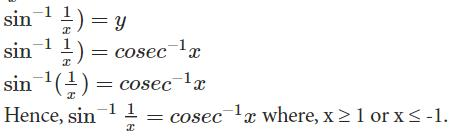Property 2

1. sin-1(-x) = – sin-1(x),    x ∈ [-1,1]
2. tan-1(-x) = -tan-1(x),   x ∈ R
3. cosec-1(-x) = -cosec-1(x), |x| ≥ 1

Proof: sin-1(-x) = -sin-1(x),    x ∈ [-1,1]
Let,  Sin-1(-x) = y

Then −x=sin y

x=−sin y

x=sin(−y)

sin−1=sin−1(sin(−y))

sin−1x=y

sin−1x=−sin−1(−x)

Hence,sin−1(−x)=−sin−1 x ∈ [-1,1]

Property 3

1. cos-1(-x) = π – cos-1 x, x ∈ [-1,1]
2. sec-1(-x) = π – sec-1x, |x| ≥ 1
3. cot-1(-x) = π – cot-1x, x ∈ R

Proof : cos-1(-x) = π – cos-1 x, x ∈ [-1,1]

Let cos−1(−x)=y

cos y=−x   x=−cos y

x=cos(π−y)

Since,  cos π−q=−cos q

cos−1 x=π−y

cos−1 x=π–cos−1–x

Hence, cos−1−x=π–cos−1x

Solved Example

Ques 1: Prove that “sin-1(-x) = – sin-1(x),    x ∈ [-1,1]”

Ans: Let, sin−1(−x)=y

Then −x=sin y

x=−sin y

x=sin(−y)

sin−1 x=arcsin(sin(−y))

sin−1 x=y

sin−1 x=−sin−1(−x)

Hence, sin−1(−x)=−sin−1 x, x ∈ [-1,1]

This concludes our discussion on the topic of trigonometric inverse functions.
Ques 2: sin-1(cos π/3)=?
Ans:
sin−1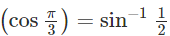[substitute cos(π/3)=1/2]
= π/6 [substitute sin-1 (1/2) = π/6]

Ques 3: Find the value of sin (π/4+Cos-1(√2/2)).
Ans:

Let y=sin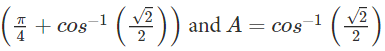Then, cosA= √2/2
Multiplying the numerator as well as denominator by √2 we get:

cosA=1/√2
A = π/4
Therefore
y = sin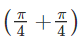y = sin (π/2)
hence, y=1.

Offer running on EduRev: Apply code STAYHOME200 to get INR 200 off on our premium plan EduRev Infinity!

## Mathematics (Maths) Class 12

209 videos|222 docs|124 tests

,

,

,

,

,

,

,

,

,

,

,

,

,

,

,

,

,

,

,

,

,

;